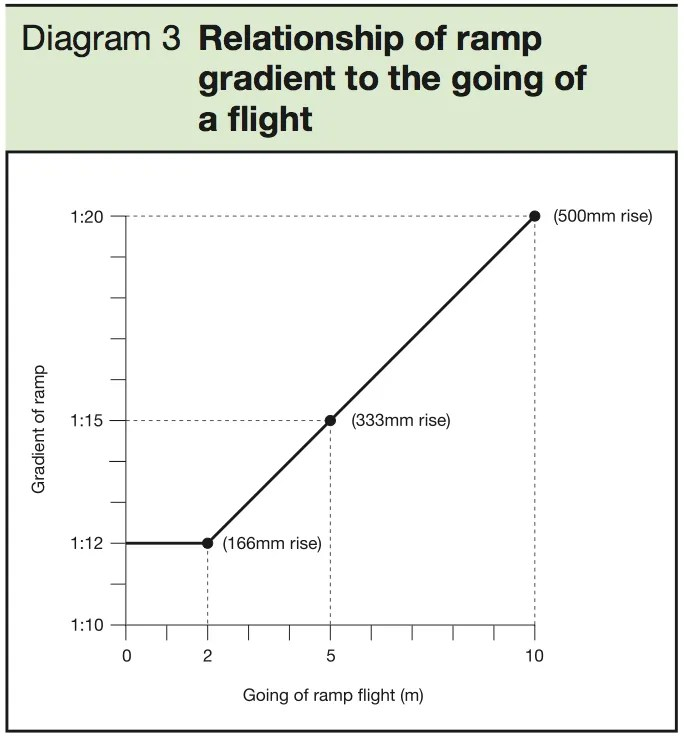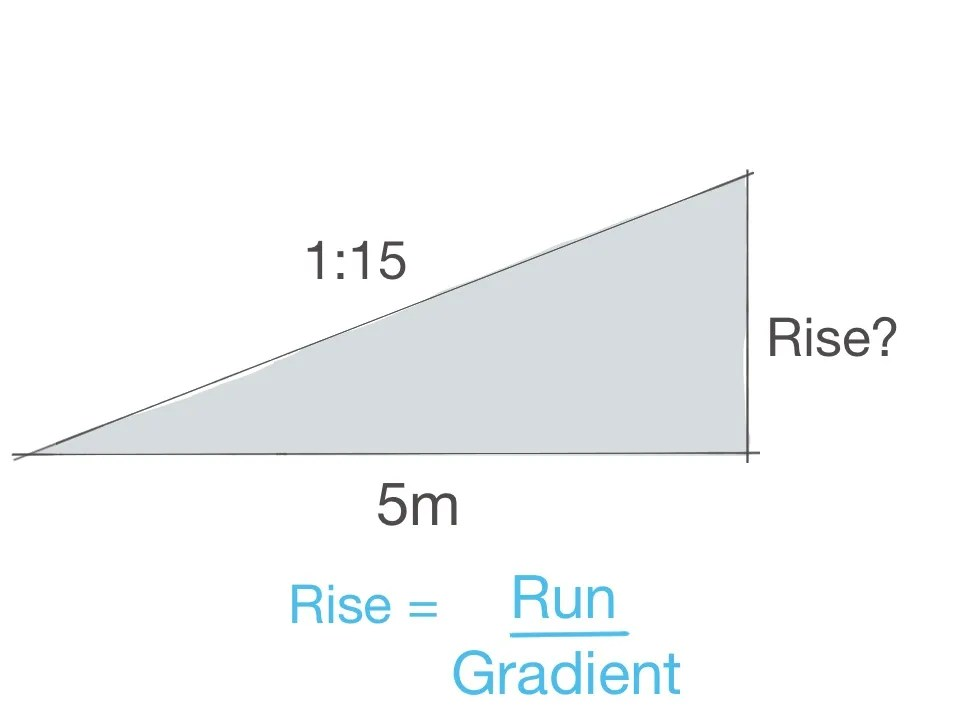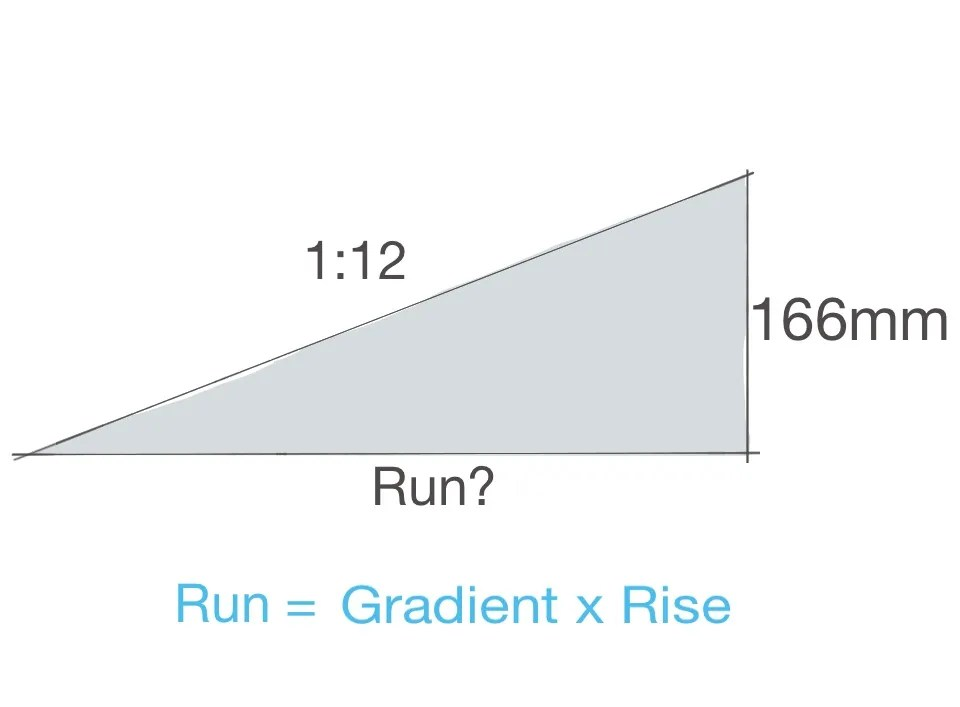# How to calculate slopes and gradients# How to calculate slopes, and gradients

You can download a pdf of this guide by clicking on the button below.

As an architect you will need to know how to calculate slopes and gradients in differing situations. The most common occurrence of this is working with ramped access to buildings, groundworks, car parks and so on. Slopes can be measured in angles, percentages and ratios. For this post I will focus on the measurement of slopes in ratios, mainly because gradient ratios are stated in the building regulations. At the end I will also take a quick look at slope percentages and how to work them out.

Approved Document Part M of the building regulations specifies limits for ramp gradients for access to a building. If access to a building is too steep it is not deemed to be a safe access and creates difficulty for people in wheelchairs who may not have the strength to propel themselves up a slope, or may have difficulty slowing down when descending a slope that is too steep. Some people find they need to stop frequently when ascending/descending ramp and therefore suitable landings are required at specified intervals to allow for this.

The table below shows the guidelines from the building regulations:To work out the gradient of an existing slope you will need the distance of the slope (run) and the height of the slope (rise). The method that follows may not be the most mathematical way to work out the ratio gradient of a slope – but it is really simple and has always worked for me!

Remember to convert units so they are the same. For example, we have a 10m ramp run and a rise of 500mm. Convert the run to 10,000mm.

## How to work out the gradientExample:

We want to work out the gradient of a ramp that has a run of 10m and a rise of 500mm.

Convert the units:

## How to work out the riseWe want to work out the rise of a ramp that has a run of 5m and a gradient of 1:15.

Rise = 5m / 15

Convert the units:

Rise = 5,000mm / 15

Rise = 333mm

## How to work out the runWe want to work out the run of a ramp that has a rise of 166mm and a gradient of 1:12.

Run = 12 x 166mm

Run = 1,992mm

Run = 1.99m (round up to 2m)

Make sure you don’t miss out on anything that is going on at First In Architecture – sign up to the newsletter now. No spam, no funny business, just useful stuff!

## How to work out slope percentageIf we want to work out the percentage of a slope, you first must ensure you convert the units so they are the same, similar to working with the ratios above.

Working on one of the previous example, lets assume we have a slope that has a run of 10m with a rise of 500mm. First convert the units.

Rise: 500mm
Run: 10,000mm

Percentage of slope = Rise / Run x 100

Percentage of slope = 500 / 10,000 x 100

Percentage of slope = 5%

I hope you find this post helpful for working out your slopes and ramps!